## Selina Concise Mathematics Class 9 ICSE Solutions Compound Interest (Without using formula)

APlusTopper.com provides step by step solutions for Selina Concise Mathematics Class 9 ICSE Solutions Chapter 2 Compound Interest (Without using formula). You can download the Selina Concise Mathematics ICSE Solutions for Class 9 with Free PDF download option. Selina Publishers Concise Mathematics for Class 9 ICSE Solutions all questions are solved and explained by expert mathematic teachers as per ICSE board guidelines.

Selina ICSE Solutions for Class 9 Maths Chapter 3 Compound Interest (Without using Formula)

Exercise 2(A)

Solution 1: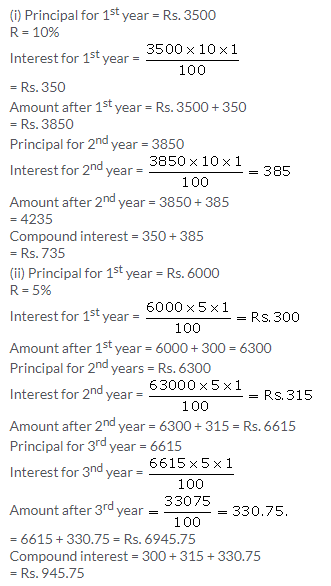Solution 2: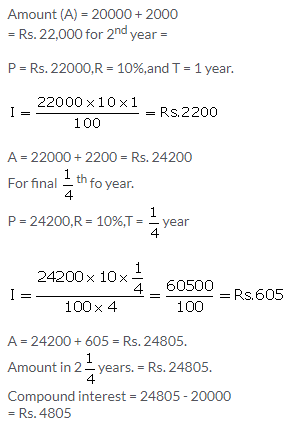Solution 3: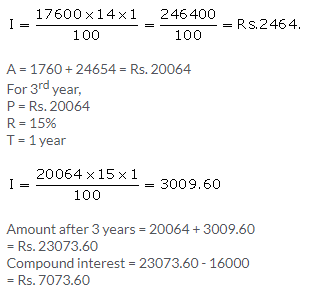Solution 4: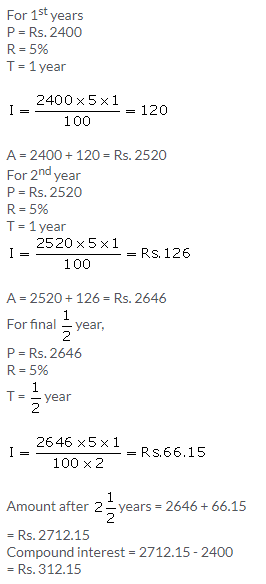Solution 5:Solution 6: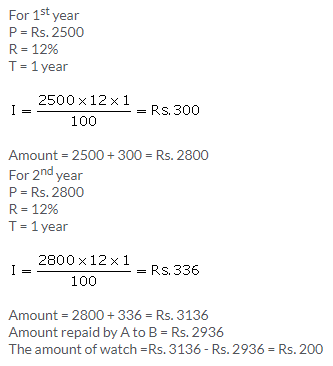Solution 7: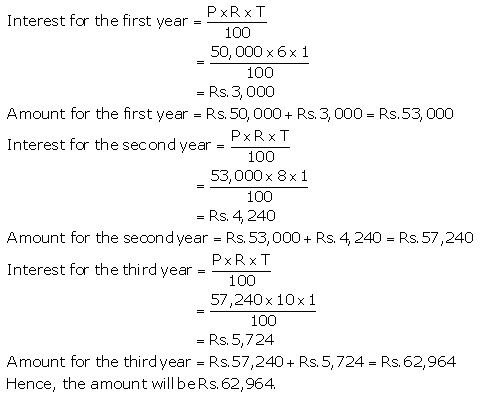Solution 8:Solution 9: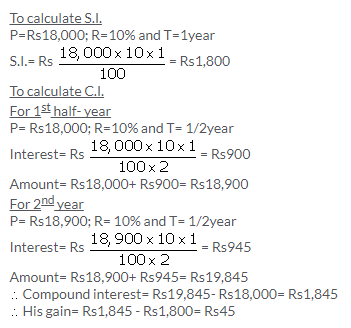Solution 10: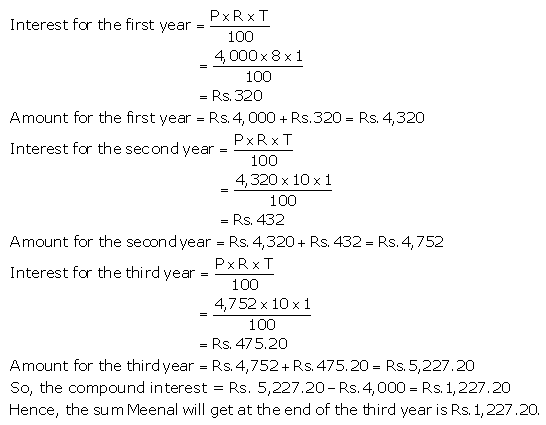Exercise 2(B)

Solution 1:Solution 2:Solution 3: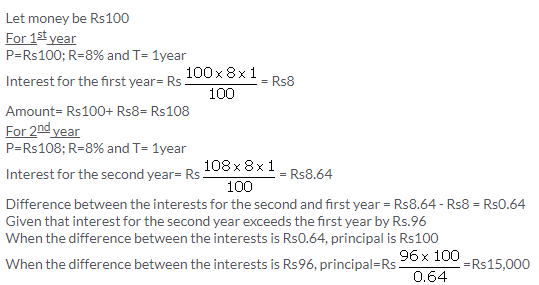Solution 4: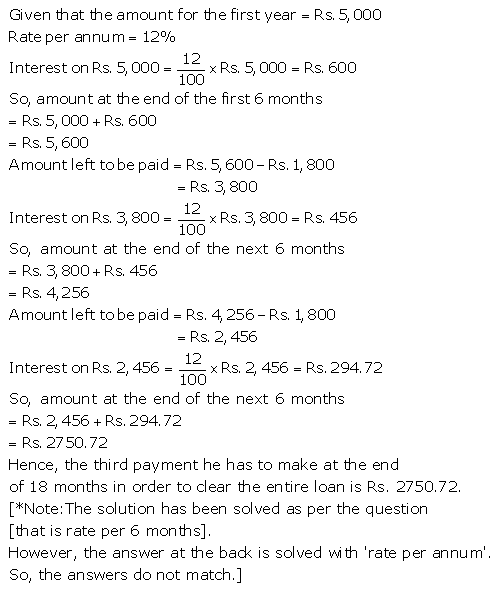Solution 5:Solution 6:Solution 7: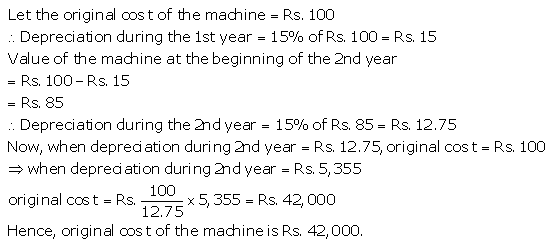Solution 8: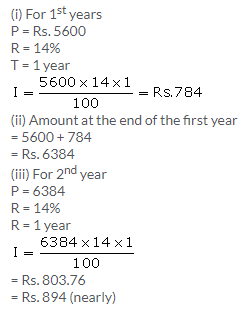Solution 9(i):Solution 9(ii):Solution 10: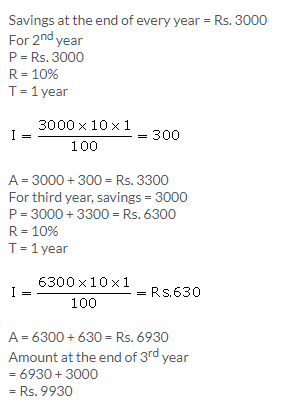Solution 11:Solution 12: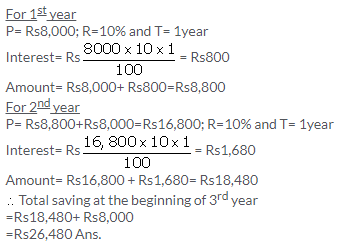Exercise 2(C)

Solution 1:Solution 2:Solution 3:Solution 4: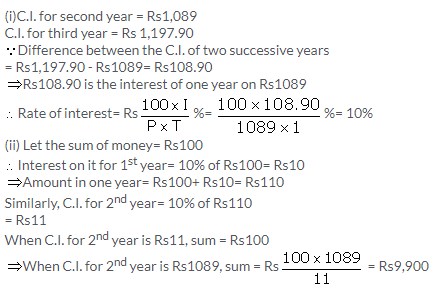Solution 5:Solution 6: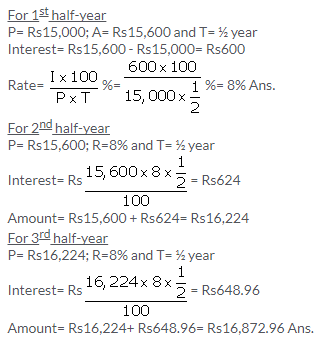Solution 7:Solution 8: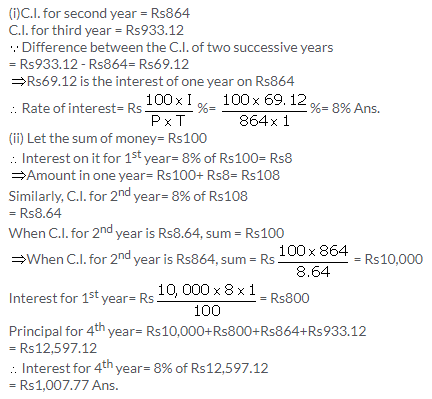Solution 9: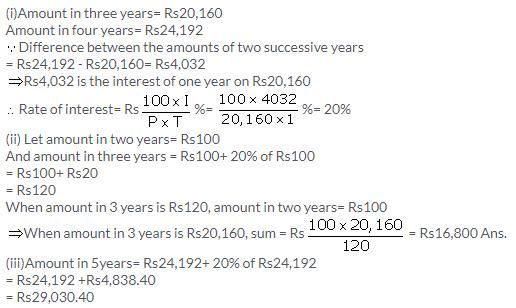Solution 10: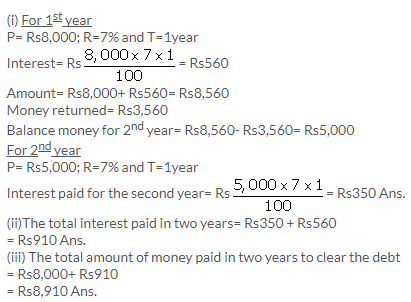Solution 11: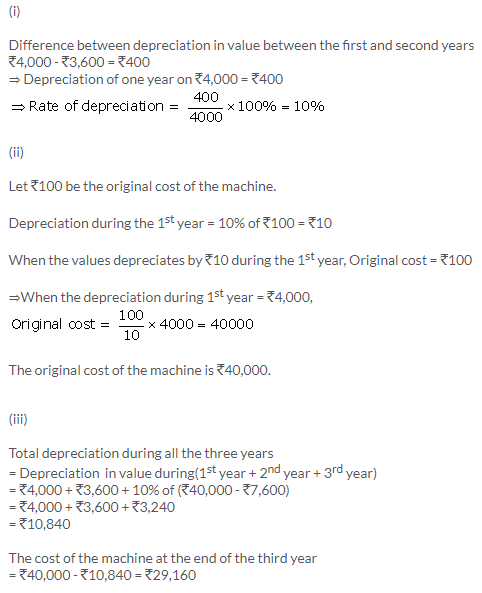Solution 12:Solution 13:Solution 14: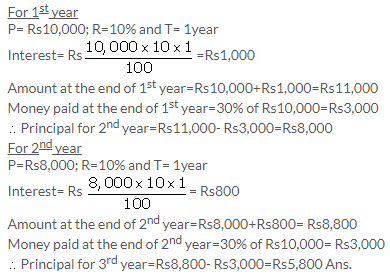Solution 15: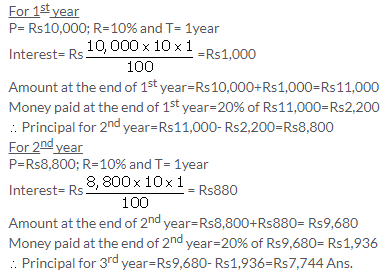Exercise 2(D)

Solution 1: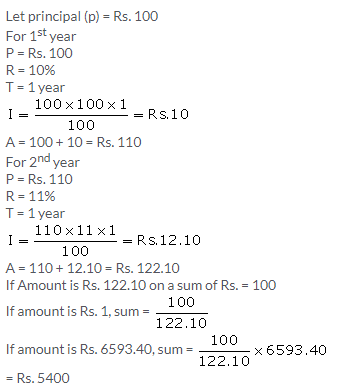Solution 2:Solution 3:Solution 4:Solution 5:Solution 6:Solution 7: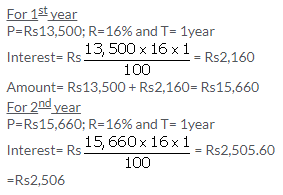Solution 8: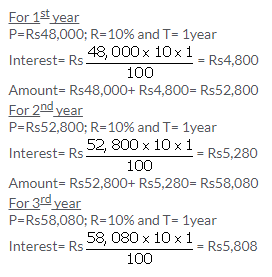Solution 9: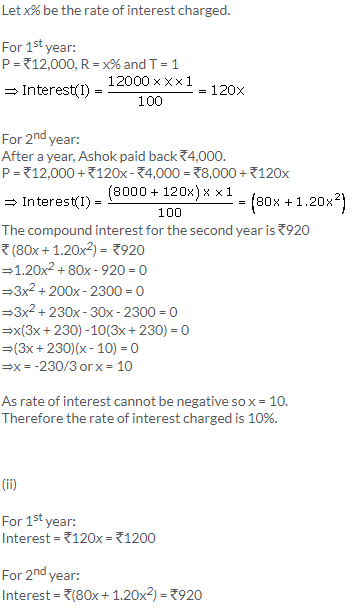The amount of debt at the end of the second year is equal to the addition of principal of the second year and interest for the two years.
Debt = Rs.8,000 + Rs.1200 + Rs.920 = Rs.10,120

Solution 10: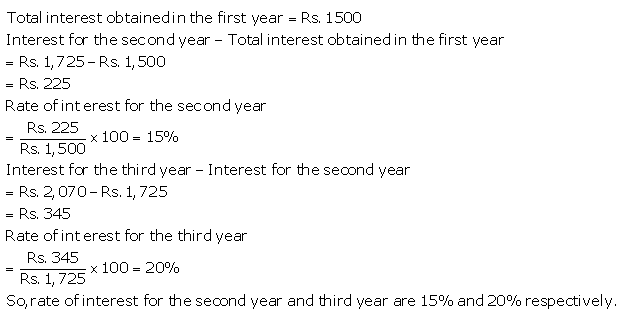More Resources for Selina Concise Class 9 ICSE Solutions